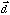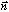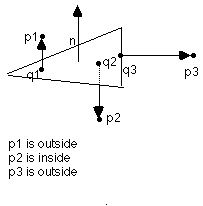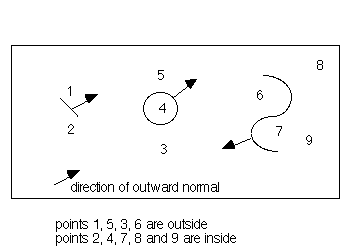# SURFACE

Defines a boundary, interface, or geometry for a selected mesh object. The release will release the previously defined surface referenced by name. All references will be removed from the geometry data structures and all constraints (icr) values associated with this surface will be removed.

## FORMAT

```surface/isurname/ ibtype / istype / surface-parameters

surface/isurname/ ibtype /sheet/cmo_name

surface/isurname/release
```

`isurname` is the name of the surface and must be unique for each surface defined by surface.

`ibtype` defines the boundary type with keywords free, intrface, reflect, intrcons or virtual. Nodes on reflect, intrcons, or virtual interfaces will be assigned node constraint (icr) values corresponding to the surfaces on which the nodes sit. The command settets will generate parent/child node chains (isn) for nodes on intrface or intrcons surfaces.

• `free` can be used for external boundaries.

• `reflect` can be used for external boundaries, assigned constraint values (icr).

• `intrface` for interior interfaces, will generate parent/child node chains (isn).

• `intrcons` for constrained internal interfaces, are assigned constraint values (icr) and will generate parent/child node chains (isn).

• `virtual` used for internal surfaces which have different materials on either side of the virtual interfaces do not separate material regions but are intended to identify other structural features of a geometry. Nodes on this surface will be assigned constraint values (icr).

`istype` are the surface parameters and are specified with the surface type in mind.

box Where xmin,ymin,zmin and xmax,ymax,zmax are the coordinates of opposite corners of a cube, i.e bottom left and top right corners.

```surface/sbox_name/ ibtype / box /xmin,ymin,zmin / xmax,ymax,zmax/
```

cylinder Where point 1 is the bottom center and point 2 is the top center of the cylinder. radius is the radius of the cylinder. Cylinders are open but finite. To create a closed cylinder cap both ends with planes.

```surface/s_name/ ibtype / cylinder x1,y1,z1 / x2,y2,z2/ radius
```

cone Where point 1 is the vertex and point 2 is the top center of the cone with radius from that point. A cone is finite but does have an open end. To create a closed cone cap the open end with a plane.

```surface/s_name/ ibtype / cone /x1,y1,z1/x2,y2,z2/radius/
```

ellipse Where point 1 is the center of the ellipsoid and point 2 is on the a semi-axis (new x), point 3 is on the b semi-axis (new y). The values ar, br, cr are radii on their respective semi-axes.

```surface/s_name/ ibtype / ellipse / x1,y1,z1 / x2,y2,z2 / x3,y3,z3 / ar, br, cr /
```

parallel (piped) Where points 1, 2, 3 are the front left, front right and back left points of the base and point 4 is the upper left point of the front face.

```surface/s_name/ ibtype / parallel / x1,y1,z1 / x2,y2,z2 / x3,y3,z3 / x4,y4,z4
```

plane Planes are infinite and their direction normal to the plane is determined by the order of the points according to the right hand rule.

```surface/s_name/ ibtype / plane or planexyz/ x1,y1,z1 / x2,y2,z2 / x3,y3,z3
surface/s_name/ ibtype / planertz / radius1, theta1, z1, radius2, theta2, z2, radius ,zcen/
surface/s_name/ ibtype / planertp radius1,theta1,phi1, radius2,theta2,phi2, radius3,theta3,phi3/ xc, yc, zc
```

sphere Where xc, yc, zc are the sphere center and radius is distance from center point to sphere surface.

```surface/s_name/ ibtype / sphere / xc, yc, zc, radius
```

sheet surfaces can be defined by cmo_name that is a 2D quad or triangle connected mesh object. See below for a description of inside/outside or left/right with respect to sheet surfaces.

```surface/s_name/ sheet / cmo_name
```

tabular (rotated tabular profile) Where point 1 and point 2 define the axis of rotation for the tabular profile with point 1 as the origin. This is followed by pairs of profile descriptors depending on the value of geom. Ifgeom is set to rz, then the r value is a radius normal to the axis of rotation and z is the distance along the new axis of rotation. If geom is set to rt then theta is the angle from the axis of rotation at point 1 and r is the distance from point 1 along theta. The first pair must start on a new line and all lines must contain pairs of data. The last pair of data must be followed by end. Note the use of & as a line continuation for the command.

```surface/s_name/ ibtype / tabular / x1,y1,z1 / x2,y2,z2 / rz rt/ &
r1,z1 &
r2,z2 &
r3,z3 &
....
rn,zn &
end

or

r1,theta1 &
r2,theta2 &
r3,theta3 &
...
rn,thetan &
end
```

## EXAMPLES

```surface/s1/release

surface / s_box / reflect / box / -1. -1. -1. / 1. 1. 1.

surface / s_cone / reflect / cone / .1 .1 .1 / .5 .5 .5 / .3

define XC     498.0
define YC     539.0
define ZBOT  -500.0
define ZTOP   500.0
define RAD     42.0
surface/ s_cyl /intrface/cylinder/XC,YC,ZBOT/XC,YC,ZTOP/ RAD

surface / sbox / reflect / ellipse / &
552250.0 4121975.0 1275.0 / &
552251.0 4121975.0 1275.0 / &
552250.0 4121976.0 1275.0 / &
500.0 225.0 125.0
region / rbox / le sbox
eltset/ereset/region/rbox

define x1 497000.
define y1 540612.
define x2 499300.
define y2 540100.
define x3 497000.
define y3 541316.
surface/SSBOX/intrface/parallel/x1,y1,1700./ &
x2,y2,1700./x3,y3,1700./x1,y1,5000./

define x1 499029.5961
define x2 500174.3616
define y1 538579.7712
define y2 539378.5612
define uleft 1779.9725
define uright 1778.7472
define lleft 1779.7481
define lright 1778.5228
surface/ swtr / intrface /plane/ x2, y1, lright / x2, y2, uright / x1, y2, uleft

surface / bbox / reflect / box / .1 .1 .1 / .9 .9 .9
surface / s1 / intrface / sheet  / cmo_2
surface / s2 / intrface / sheet  / cmo_3
region / r1 / lt bbox and gt s1 and gt s2
region / r2 / lt bbox and gt s1 and lt s2
region / r3 / lt bbox and lt s1 and gt s2
region / r4 / lt bbox and lt s1 and lt s2

```

### inside/outside with respect to sheet surfaces

Inside/outside will be determined by the following algorithm:

• For the point being considered, p, find the nearest sheet triangle and the closest point, q, to p that lies on that triangle.

• Construct the vector, from q to p.

• Construct the outward normal to the triangle,The outward normal is constructed using the right hand rule and the order of the points in the sheet. Sheets may be specified as quad Mesh Object (i.e. a 2 dimensional array of points containing the coordinates of the corners of each quad). Either two triangles (divide each quad in two using point (i,j) and (i+1,j+1)) or four triangles (add a point in the center of the quad) are generated by each quad. Applying the right hand rule to the points (i,j), (i+1,j), (i+1,j+1) gives the direction of the normal for all triangles created from the quad.

• If< 0 then the point is inside. If>0 the point is outside. If* n = 0, and if p is on the triangle then p=q and p in on the triangle.

• If= 0 and p is not on the triangle then p is outside.One implication of this definition is that the concept of shadows cast by open sheets no longer is valid. Sheets may be considered to extend to the boundary of the geometry.